On a recent visit to Cape May, New Jersey I bought and read Shark Attacks of the Jersey Shore: A History, which is an interesting account of verified shark attacks in New Jersey since the nineteenth century.While I was reading the book, I grew curious about modeling the frequency of shark attacks and went on the hunt for relevant data. There have not been many recent shark attacks in New Jersey, so I broadened my search and found the Global Shark Attack File (GSAF). The GSAF provides a fairly detailed incident log which appears to be updated every few days. This post presents an analysis of the GSAF data in Python using PyMC3. (It is worth mentioning that I am not a shark biologist, and I am sure specialists have produced much more useful and accurate models of shark attacks. Still, this seems like a fun small project as long as we don’t take the results too seriously.)

First we make some Python imports and do a bit of housekeeping.

%matplotlib inline
from functools import reduce
from warnings import filterwarnings
from aesara import tensor as at
import arviz as az
from matplotlib import pyplot as plt
import numpy as np
import pandas as pd
import pymc3 as pm
import seaborn as sns
import us
You are running the v4 development version of PyMC3 which currently still lacks key features. You probably want to use the stable v3 instead which you can either install via conda or find on the v3 GitHub branch: https://github.com/pymc-devs/pymc3/tree/v3
filterwarnings('ignore', category=UserWarning, module='aesara')
filterwarnings('ignore', category=UserWarning, module='arviz')
filterwarnings('ignore', category=UserWarning, module='pandas')
plt.rcParams['figure.figsize'] = (8, 6)
sns.set(color_codes=True)

## Load and Transform the Data

%%bash
DATA_DEST=./data/GSAF5.xls

if [[ ! -e $DATA_DEST ]]; then wget -q -O$DATA_DEST DATA_URL fi full_df = pd.read_excel('./data/GSAF5.xls', usecols=[ "Case Number", "Date", "Type", "Country", "Area", "Location", "Fatal (Y/N)" ], index_col="Case Number") full_df["Date"] = full_df["Date"].apply(pd.to_datetime, errors='coerce') full_df.head() Date Type Country Area Location Fatal (Y/N) Case Number 2021.06.14.b 2021-06-14 Unprovoked USA Florida New Smyrna Beach, Volusia County N 2021.06.14.a 2021-06-14 Unprovoked USA Florida New Smyrna Beach, Volusia County N 2021.06.12 2021-06-12 Provoked ENGLAND West Sussex Littlehampton N 2021.06.11 2021-06-11 Unprovoked AUSTRALIA Western Australia Five Fingers Reef N 2021.05.23 2021-05-23 Unprovoked USA South Carolina Burkes Beach, Hilton Head, Beaufort County N full_df.tail() Date Type Country Area Location Fatal (Y/N) Case Number NaN NaT NaN NaN NaN NaN NaN NaN NaT NaN NaN NaN NaN NaN NaN NaT NaN NaN NaN NaN NaN NaN NaT NaN NaN NaN NaN NaN xx NaT NaN NaN NaN NaN NaN We can already see by inspecting the data frame that there will be quite a bit of missing data to handle. full_df.index.isnull().mean() 0.6595373706846449 full_df.isnull().mean() Date 0.775621 Type 0.742880 Country 0.744391 Area 0.760587 Location 0.763726 Fatal (Y/N) 0.763842 dtype: float64 We begin by filtering out rows with missing data in important columns. FILTERS = [ ~full_df.index.isnull(), ~full_df["Date"].isnull(), ~full_df["Type"].isnull() ] (full_df[reduce(np.logical_and, FILTERS)] .isnull() .mean()) Date 0.000000 Type 0.000000 Country 0.004843 Area 0.052413 Location 0.061927 Fatal (Y/N) 0.079398 dtype: float64 We see now that there is significantly less missing data, so we proceed to examine various aspects of the data. ax = (full_df[reduce(np.logical_and, FILTERS)] ["Type"] .value_counts() .plot(kind='barh')) ax.set_xscale('log'); ax.set_xlabel("Number of attacks"); ax.invert_yaxis(); ax.set_ylabel("Type of attack");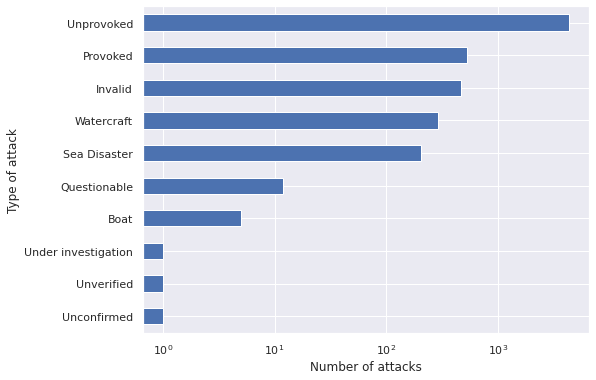Unprovoked attacks are by far the most common. We will focus our analysis on this type of attack. FILTERS.append(full_df["Type"] == "Unprovoked") ax = (full_df[reduce(np.logical_and, FILTERS)] ["Country"] .value_counts() .plot(kind='barh', figsize=(8, 30))) ax.set_xscale('log'); ax.set_xlabel("Number of unprovoked attacks"); ax.invert_yaxis(); ax.set_ylabel("Country");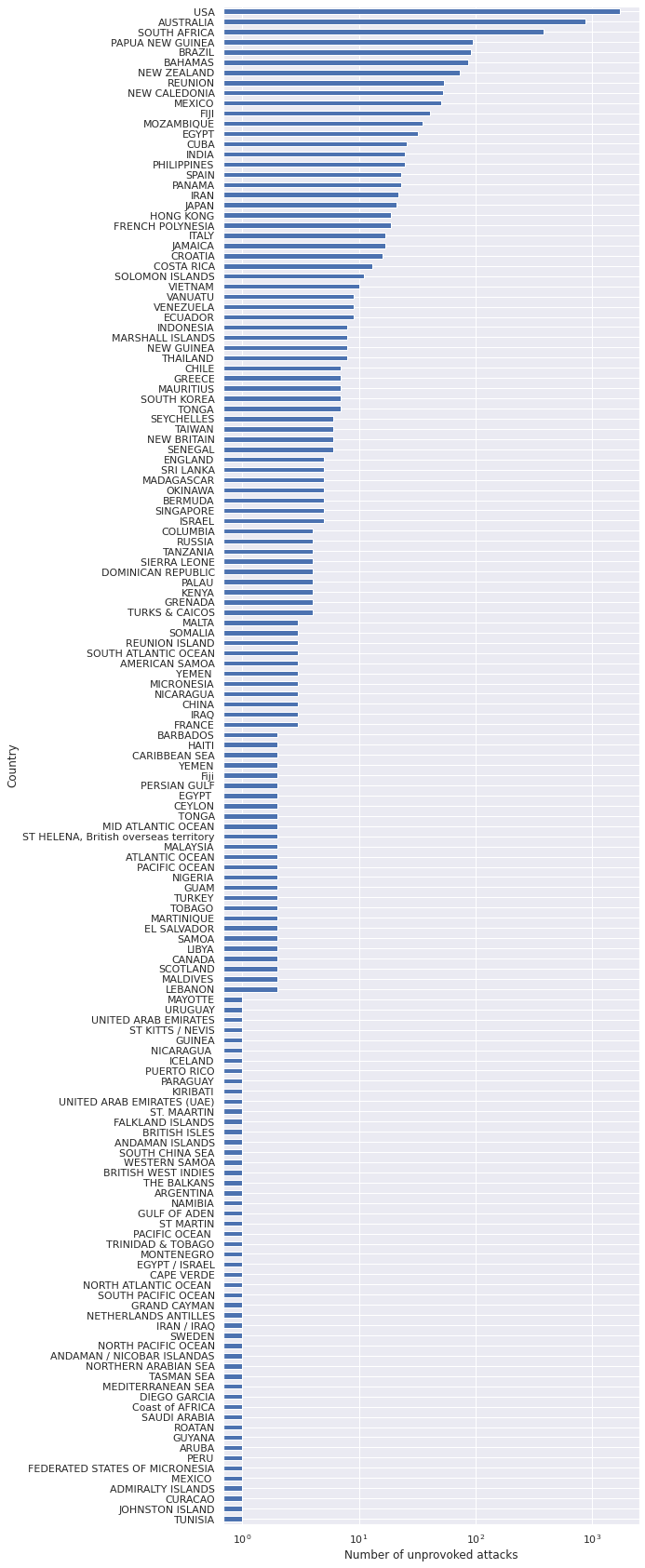While the data contain information about attacks in many countries, we will focus on the United States. This focus is due partially to the fact that I was in New Jersey when considering this problem, and partially due to the fact that finding standardized data across countries can be more challenging than within different regions of a single country. FILTERS.append(full_df["Country"] == "USA") ax = (full_df[reduce(np.logical_and, FILTERS)] ["Area"] .value_counts() .plot(kind='barh', figsize=(8, 12))) ax.set_xscale('log'); ax.set_xlabel("Number of unprovoked attacks"); ax.invert_yaxis(); ax.set_ylabel("Country");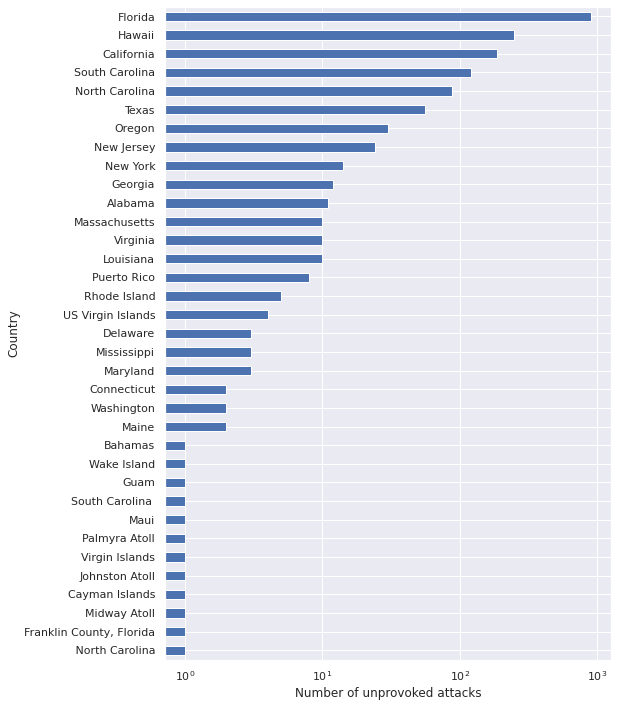We see that for unprovoked attacks in the United States, Area roughly corresponds to state, with some territories included as well. Since most of the territories appear very rarely, we restrict our analysis to states so that it is easier to find information about them. FILTERS.append( full_df["Area"].isin([ state.name for state in us.states.STATES ]) ) Finally we look at how the number of shark attacks has changed over time. ax = (full_df.assign(Year=full_df["Date"].dt.year) [reduce(np.logical_and, FILTERS)] ["Year"] .value_counts() .sort_index() .plot()) ax.set_xlabel("Year"); ax.set_ylabel("Unprovoked shark attacks\nin the United States");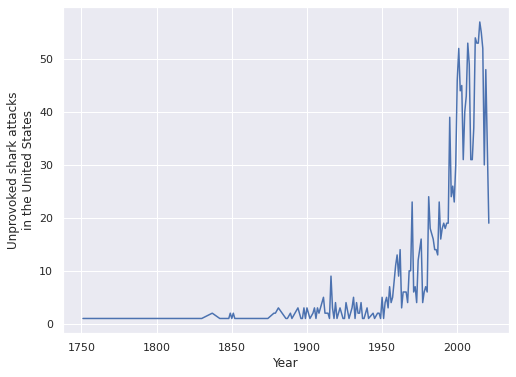We see that the number of shark attacks has increased over time. This phenomenon is likely partially due to population growth and partially due to improved reporting mechanisms for shark attacks. We willk keep a relatively modern focus an analysze shark attacks between 1960 and 2020. YEAR_RANGE = (1960, 2020) FILTERS.append(full_df["Date"].dt.year.between(*YEAR_RANGE)) Now that we have defined the set of attacks we will model, we produce another data frame including only these attacks and make some light transformations of the data. df = (full_df[reduce(np.logical_and, FILTERS)] .copy() .rename(columns={"Area": "State"})) df["Year"] = df["Date"].dt.year df.head() Date Type Country State Location Fatal (Y/N) Year Case Number 2020.12.30 2020-12-30 Unprovoked USA California Coronado, San Diego County N 2020 2020.12.08 2020-12-08 Unprovoked USA Hawaii Honolua Bay Y 2020 2020.12.06.b 2020-12-06 Unprovoked USA Oregon Seaside Cove, Clatsop County N 2020 2020.11.26 2020-11-26 Unprovoked USA Hawaii Maui N 2020 2020.10.31 2020-10-31 Unprovoked USA Florida Ormond Beach N 2020 df.tail() Date Type Country State Location Fatal (Y/N) Year Case Number 1904.00.00.a 1970-01-01 00:00:00.000001904 Unprovoked USA Hawaii Off Diamond Head, Honolulu, O’ahu Y 1970 1896.00.00.b 1970-01-01 00:00:00.000001896 Unprovoked USA Florida NaN Y 1970 1883.00.00.a 1970-01-01 00:00:00.000001883 Unprovoked USA South Carolina NaN Y 1970 1882.00.00.b 1970-01-01 00:00:00.000001882 Unprovoked USA Florida In the bay near the naval yard at Pensacola, E… N 1970 1852.00.00 1970-01-01 00:00:00.000001852 Unprovoked USA South Carolina Mount Pleasant, Charleston County Y 1970 df.shape (1514, 7) After applying these filters, just over 1,500 attacks remain. This analysis will focus on the number of shark attacks in a state in a given year. Subsequent posts may analyze other aspects of this data. First we count the number of attacks in a given state in a given year. (We will shorten the phrase “unprovoked shark attacks in the United States between 1960 and 2020” to “attacks” for the remainder of the post.) attacks_nz = (df.groupby(["State", "Year"]) .size() .rename("Attacks")) attacks_nz.describe() count 333.000000 mean 4.546547 std 6.527174 min 1.000000 25% 1.000000 50% 2.000000 75% 5.000000 max 38.000000 Name: Attacks, dtype: float64 The series attacks_nz includes a row only when there was at least one attack in that state in that year. We also want to include zero entries for state/year combinations that saw now attacks, which we do now by reindexing attacks_nz. attacks_index = (pd.MultiIndex.from_product(( attacks_nz.index .get_level_values("State") .unique(), YEAR_RANGE + np.arange(attacks_nz.index .get_level_values("Year") .values .ptp()))) .rename("Year", level=1)) attacks_df = (attacks_nz.reindex(attacks_index, fill_value=0) .astype(np.int64) .to_frame()) attacks_df.head() Attacks State Year Alabama 1960 0 1961 0 1962 0 1963 0 1964 0 attacks_df.tail() Attacks State Year Washington 2015 0 2016 0 2017 1 2018 0 2019 0 ## Modeling We now turn to modeling the data. ax = attacks_df["Attacks"].hist(bins=attacks_df["Attacks"].max() + 1) ax.set_xlabel("Number of attacks"); ax.set_yscale('log'); ax.set_ylabel("Number of state-years");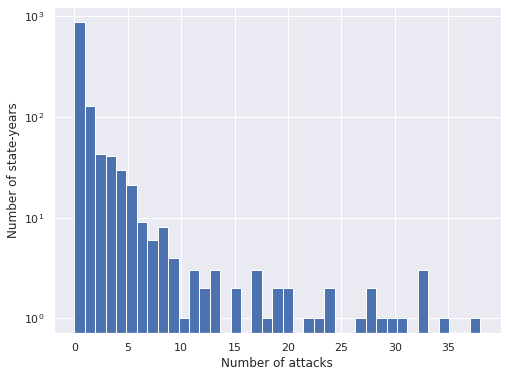We see that the vast majority of state-years have no shark attacks, and that when there is at least one attack, there are rarely very many. We see that the index of dispersion is significantly larger than one, so the data shows overdispersion. attacks_df.var() / attacks_df.mean() Attacks 12.729978 dtype: float64 ### Negative Binomial Due to this overdispersion, we begin with a Negative Binomial model. Let $$y_{i, t}$$ represent the number of attacks in the $$i$$-th state in year $$t$$. We use the priors \begin{align*} \mu & \sim \operatorname{Lognormal}(0, 2.5^2) \\ \alpha & \sim \operatorname{Half}-N(2.5^2). \end{align*} with pm.Model() as nb_model: μ = pm.Lognormal("μ", 0., 2.5) α = pm.HalfNormal("α", 2.5) We now let $$y_{i, t} \sim NB(\mu, \alpha)$$ y = attacks_df["Attacks"].values with nb_model: obs = pm.NegativeBinomial("obs", μ, α, observed=y) We use pymc3 to sample from the posterior distribution of this model. CHAINS = 3 SEED = 12345 SAMPLE_KWARGS = { 'cores': CHAINS, 'random_seed': [SEED + i for i in range(CHAINS)], 'return_inferencedata': True } with nb_model: nb_trace = pm.sample(**SAMPLE_KWARGS) Auto-assigning NUTS sampler... Initializing NUTS using jitter+adapt_diag... Multiprocess sampling (3 chains in 3 jobs) NUTS: [μ, α] 100.00% [6000/6000 00:15<00:00 Sampling 3 chains, 0 divergences] Sampling 3 chains for 1_000 tune and 1_000 draw iterations (3_000 + 3_000 draws total) took 20 seconds. The standard sampling diagnostics (energy plots, BFMI, and $$\hat{R}$$) show no cause for concern. def make_diagnostic_plots(trace, axes=None, min_mult=0.995, max_mult=1.005): if axes is None: fig, axes = plt.subplots(ncols=2, sharex=False, sharey=False, figsize=(16, 6)) az.plot_energy(trace, ax=axes) rhat = az.rhat(trace).max() axes.barh(np.arange(len(rhat.variables)), rhat.to_array(), tick_label=list(rhat.variables.keys())) axes.axvline(1, c='k', ls='--') axes.set_xlim( min_mult * min(rhat.min().to_array().min(), 1), max_mult * max(rhat.max().to_array().max(), 1) ) axes.set_xlabel(r"\hat{R}\$")

axes.set_ylabel("Variable")

return fig, axes
make_diagnostic_plots(nb_trace);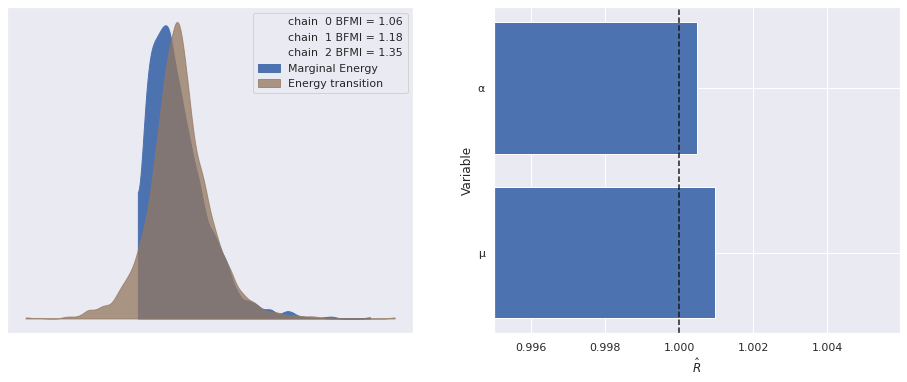Examining the posterior distribution for $$\mu$$ shows that the model will predicts more than one shark attack per year on average.

az.plot_posterior(nb_trace, var_names=["μ"]);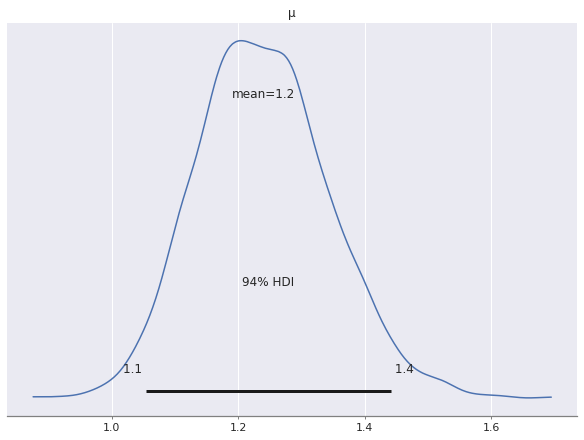This prediction of about one shark attack per state per year captures nationwide average well enough, but is clearly not very useful at the state level. We see specifically how bad it is by examining the posterior predictions of the model.

with nb_model:
nb_pp_trace = pm.sample_posterior_predictive(nb_trace)

100.00% [3000/3000 00:05<00:00]

First we produce a long data frame where each row represents a single posterior predictive sample from the distribution of the number of attacks in a given state-year. This data frame will be the basis for all of our posterior predictive plots.

def make_pp_full_df(pp_obs):
return (pd.DataFrame(
{i: samples for i, samples in enumerate(pp_obs)},
index=attacks_df.index
)
.reset_index()
.melt(id_vars=["State", "Year"],
var_name="Sample")
.set_index(["State", "Year", "Sample"]))
nb_pp_full_df = make_pp_full_df(nb_pp_trace["obs"])
nb_pp_full_df.head()
value
State Year Sample
Alabama 1960 0 1
1961 0 0
1962 0 0
1963 0 0
1964 0 0

ALPHA = 0.05
def summarize_pp_df(pp_full_df, level=None, alpha=ALPHA):
if level is None:
level = ["State", "Year"]

return (pp_full_df.groupby(level=level)
["value"]
.agg(
mean='mean',
sum='sum',
low=lambda s: s.quantile(alpha / 2.),
high=lambda s: s.quantile(1. - alpha / 2.),
)
.assign(attacks=attacks_df["Attacks"]
.groupby(level=level)
.sum()))
nb_pp_df = summarize_pp_df(nb_pp_full_df)
nb_pp_df.head()
mean sum low high attacks
State Year
Alabama 1960 1.231000 3693 0 12.000 0
1961 1.212667 3638 0 12.000 0
1962 1.206000 3618 0 11.025 0
1963 1.232000 3696 0 12.000 0
1964 1.177667 3533 0 11.000 0

The data frame nb_pp_df contains the posterior predictive mean and posterior predictive quantiles for each state-year combination. We now plot the predictions and actual data, broken down by state.

def plot_pp_over_time(data=None, y="mean", *args, **kwargs):
pp_df = data

ax = plt.gca()
(pp_df.plot("Year", y,
c='k', label="Posterior expected value",
ax=ax))
(pp_df.reset_index()
.plot("Year", "attacks",
kind='scatter', c='k', zorder=5,
label="Actual", ax=ax))

ax.fill_between(pp_df["Year"], pp_df["low"], pp_df["high"],
color='C0', alpha=0.5,
label="95% posterior credible interval")

ax.set_ylabel("Number of shark attacks")

return ax
grid = sns.FacetGrid(nb_pp_df.reset_index(), col="State",
col_wrap=2, sharey=False, aspect=1.5)
grid.map_dataframe(plot_pp_over_time);

grid.axes.legend(loc="upper left");
grid.set_titles("{col_name}");
grid.fig.tight_layout();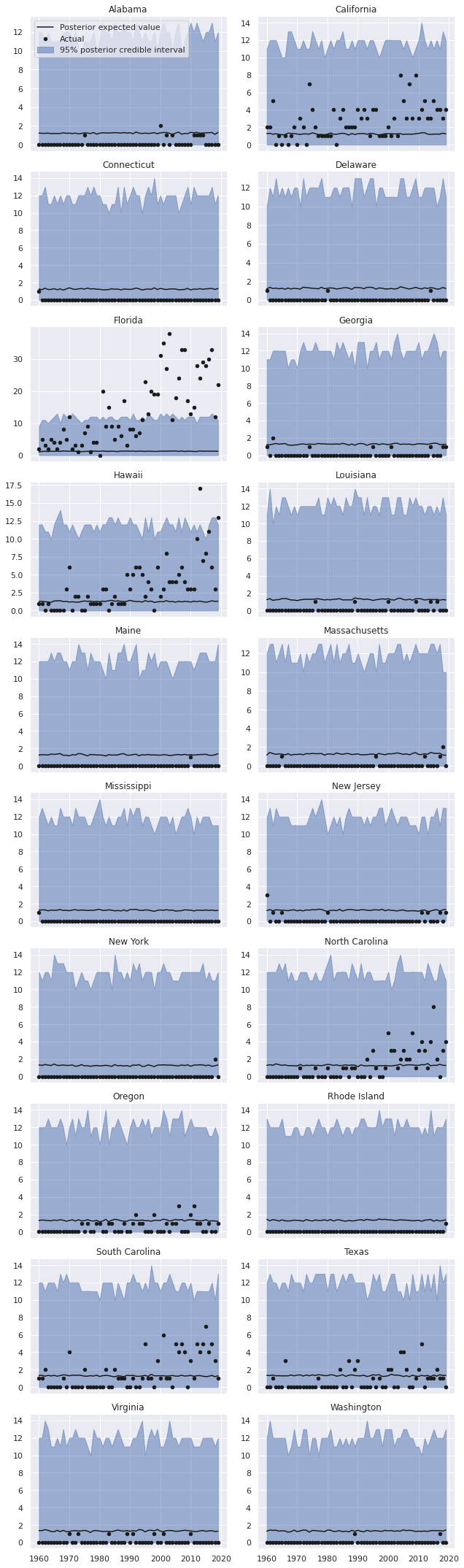We see that the predictions for each state are indeed the same (ignoring some slight Monte Carlo variation), and therefore vastly overpredict shark attacks for most states while massively underpredicting shark attacks for some states.

The plot below which does not include a time axis further reinforces this point.

nb_pp_state_df = summarize_pp_df(nb_pp_full_df, level="State")
ax = (nb_pp_state_df.reset_index()
.plot.scatter("mean", "State", color='C0',
xerr=nb_pp_state_df[["low", "high"]]
.sub(nb_pp_state_df["mean"],
axis=0)
.abs()
.values.T,
zorder=5,
label="Posterior predictive mean"))
(attacks_df.reset_index()
.plot.scatter("Attacks", "State",
color='k', alpha=0.5,
label="Actual", ax=ax));

ax.set_xlabel("Number of shark Attacks");
ax.invert_yaxis();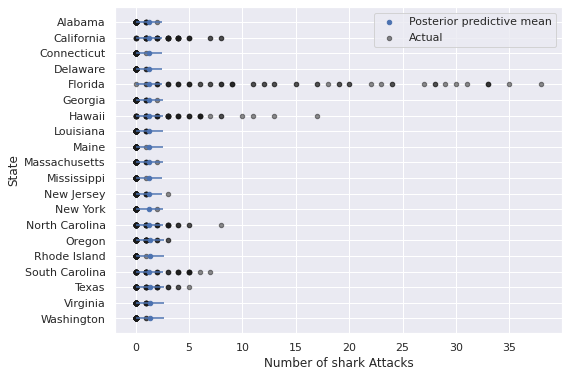The predictions from this model are particularly bad for states like California, Florida, Hawaii, and the Carolinas, where years with many shark attacks are relatively more common than they are for other states.

### Negative Binomial Regression

California, Florida, and to a lesser extent the Carolinas are similar in that they have relatively large populations. All of these states are similar in that their population is relatively concentrated on the coast.

The table below shows that overdispersion is still quite prevalent when we condition on state, so will use negative binomial regression to account for the effect of state-level factors on the number of attacks.

(attacks_df.groupby(level="State")
.var()
.div(attacks_df.groupby("State")
.mean()))
Attacks
State
Alabama 1.090395
California 1.408464
Connecticut 1.000000
Delaware 0.966102
Florida 8.373881
Georgia 1.090395
Hawaii 3.457544
Louisiana 0.915254
Maine 1.000000
Massachusetts 1.254237
Mississippi 1.000000
New Jersey 1.457627
New York 2.000000
North Carolina 2.342930
Oregon 1.156634
Rhode Island 1.000000
South Carolina 2.382694
Texas 1.917465
Virginia 0.881356
Washington 0.983051

First we load state-leve population and coastline data from Wikipedia.

POP_URL = "https://en.wikipedia.org/wiki/List_of_U.S._states_and_territories_by_historical_population"

.iloc[:-1]
.melt(id_vars="Name",
var_name="Year",
value_name="Population")
.rename(columns={"Name": "State"})
.fillna(0)
.astype({
"Year": np.int64,
"Population": np.float64
})
.set_index(["State", "Year"])
.sort_index()
["Population"])
population.head()
State    Year
Alabama  1960    3266740.0
1970    3444165.0
1980    3893888.0
1990    4040587.0
2000    4447100.0
Name: Population, dtype: float64
population.tail()
State    Year
Wyoming  1980    469557.0
1990    453588.0
2000    493782.0
2010    563626.0
2020    576851.0
Name: Population, dtype: float64

The series population contains the population of each state according to the United States census conducted every ten years.

COAST_URL = "https://en.wikipedia.org/wiki/List_of_U.S._states_and_territories_by_coastline"

coast_df = (coast_df[["State or territory", "Method 1 (CRS)", "Coast/area ratio (ft/mi2)"]]
.iloc[:-1])
coast_df.columns = coast_df.columns.droplevel(0)
coast_df = coast_df.drop(["Rank", "Method 2"], axis=1)
coast_df = (coast_df.rename(columns={
"State or territory": "State",
"Method 1": "Coastline to area"
})
.set_index("State")
.sort_index())
coast_df.head()
Coastline Coastline to area
State
Alabama 53 mi (85 km) 5.3
Alaska 6,640 mi (10,690 km) 53
American Samoa
California 840 mi (1,350 km) 27
Connecticut 96 mi (154 km) 91

coast_df["Coastline"] = (
coast_df["Coastline"]
.str.split(expand=True)
.iloc[:, 0]
.str.replace(",", "")
.str.replace("[–—]", "0", regex=True)
.astype(np.float64)
)
coast_df["Coastline to area"] = (
coast_df["Coastline to area"]
.astype(str)
.str.replace("[–—]", "-1", regex=True)
.astype(np.float64)
.replace(-1, np.nan)
)
coast_df.head()
Coastline Coastline to area
State
Alabama 53.0 5.3
American Samoa 0.0 NaN
California 840.0 27.0
Connecticut 96.0 91.0

coast_df.tail()
Coastline Coastline to area
State
U.S. Minor Outlying Islands 0.0 NaN
U.S. Virgin Islands 0.0 NaN
Virginia 112.0 14.0
Washington 157.0 12.0
Wisconsin 0.0 NaN

The data frame coast_df contains the length of a state’s coastline (in miles) as well as the ratio of its coastline to area (in square miles).

We now combine attacks, population, and coast_df into a single data frame.

attacks_df = (attacks_df.merge(coast_df,
left_index=True, right_index=True)
.merge(population, how='left',
left_index=True, right_index=True)
.fillna(method='ffill'))
attacks_df["Population to coastline"] = attacks_df["Population"] / attacks_df["Coastline"]
attacks_df.head()
Attacks Coastline Coastline to area Population Population to coastline
State Year
Alabama 1960 0 53.0 5.3 3266740.0 61636.603774
1961 0 53.0 5.3 3266740.0 61636.603774
1962 0 53.0 5.3 3266740.0 61636.603774
1963 0 53.0 5.3 3266740.0 61636.603774
1964 0 53.0 5.3 3266740.0 61636.603774

attacks_df.tail()
Attacks Coastline Coastline to area Population Population to coastline
State Year
Washington 2015 0 157.0 12.0 6724540.0 42831.464968
2016 0 157.0 12.0 6724540.0 42831.464968
2017 1 157.0 12.0 6724540.0 42831.464968
2018 0 157.0 12.0 6724540.0 42831.464968
2019 0 157.0 12.0 6724540.0 42831.464968

Here the population data is from the most recent United States census prior to the year in question (thanks to fillna(method='ffill'). Below we plot the relationship between the four explanatory variables and the number of attacks.

fig, axes = plt.subplots(ncols=2, nrows=2, sharey=True,
figsize=(12, 9))

for col, ax in zip(attacks_df.columns[1:], axes.ravel()):
attacks_df.plot.scatter(col, "Attacks",
color='C0', alpha=0.5,
ax=ax);

ax.set_xscale('log');
ax.set_yscale('log');

fig.tight_layout();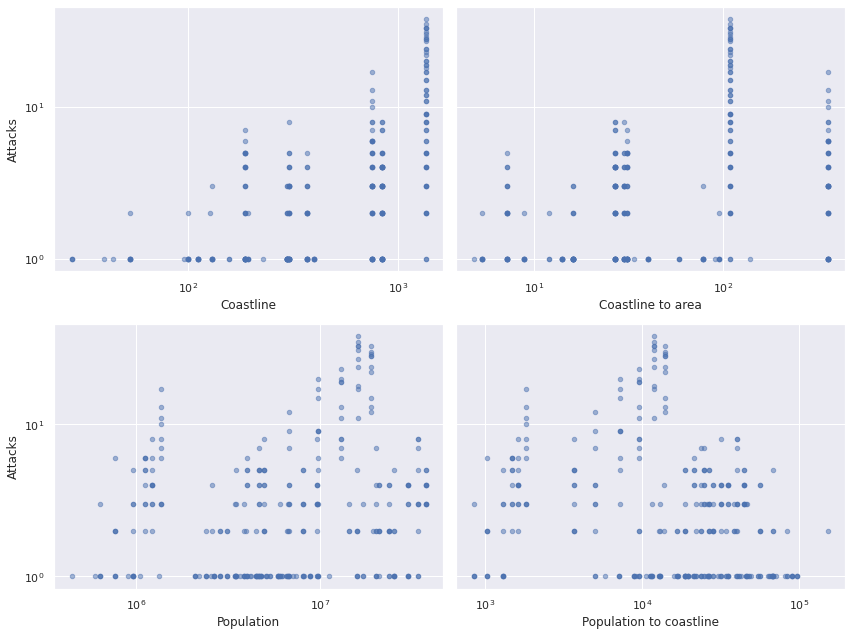The top two and bottom two plots are very similar. We choose to use coastline length (in miles) and population as our predictors since those relationships seem to be a bit more linear on the log-log scale.

We standardize the logarithms of coastline length and population before using them as predictors.

def standardize(x):
return (x - x.mean()) / x.std()
log_coast = np.log(attacks_df["Coastline"].values)
x_coast = at.constant(standardize(log_coast))
log_pop = np.log(attacks_df["Population"].values)
x_pop = at.constant(standardize(log_pop))

We use the priors $$\beta_0, \beta_{\text{coast}}, \beta_{\text{pop}} \sim N(0, 2.5^2)$$ on the regression coefficients and set

$\eta_{i, t} = \beta_0 + \beta_{\text{coast}} \cdot x_{\text{coast}, i} + \beta_{\text{pop}} \cdot x_{\text{pop}, i, t}.$ The mean is then $$\mu_{i, t} = \exp \eta_{i, t}.$$

with pm.Model() as nb_reg_model:
β0 = pm.Normal("β0", 0., 2.5)
β_coast = pm.Normal("β_coast", 0., 2.5)
β_pop = pm.Normal("β_pop", 0., 2.5)
η = β0 + β_coast * x_coast + β_pop * x_pop
μ = at.exp(η)

As in the previous model, $$\alpha \sim \operatorname{Half}-N(2.5^2)$$ and $$y_{i, t} \sim NB(\mu_{i, t}, \alpha)$$.

with nb_reg_model:
α = pm.HalfNormal("α", 2.5)
obs = pm.NegativeBinomial("obs", μ, α, observed=y)

We again sample from the posterior distribution of the model.

with nb_reg_model:
nb_reg_trace = pm.sample(**SAMPLE_KWARGS)
Auto-assigning NUTS sampler...
Multiprocess sampling (3 chains in 3 jobs)
NUTS: [β0, β_coast, β_pop, α]

100.00% [6000/6000 00:24<00:00 Sampling 3 chains, 0 divergences]

Sampling 3 chains for 1_000 tune and 1_000 draw iterations (3_000 + 3_000 draws total) took 25 seconds.

As before, the standard sampling diagnostics show no cause for concern.

make_diagnostic_plots(nb_reg_trace);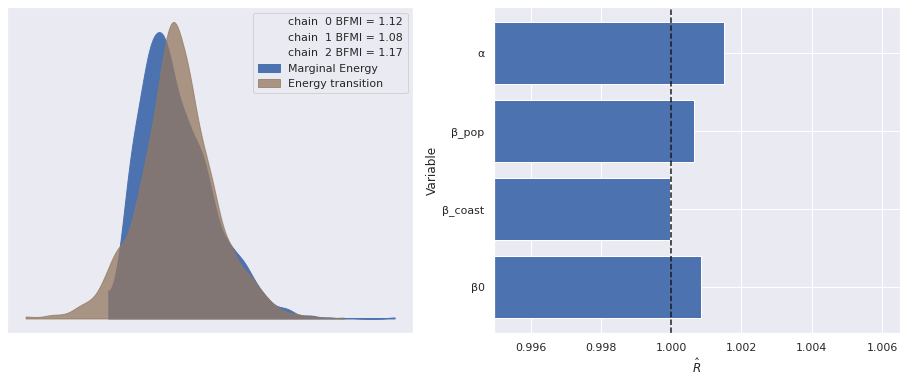To see if the inclusion of these predictors has improved the model fit, we sample from and plot the posterior predictive distributions per state-year.

with nb_reg_model:
nb_reg_pp_trace = pm.sample_posterior_predictive(nb_reg_trace)

100.00% [3000/3000 00:02<00:00]

nb_reg_pp_full_df = make_pp_full_df(nb_reg_pp_trace["obs"])
nb_reg_pp_df = summarize_pp_df(nb_reg_pp_full_df)
grid = sns.FacetGrid(nb_reg_pp_df.reset_index(), col="State",
col_wrap=2, sharey=False, aspect=1.5)
grid.map_dataframe(plot_pp_over_time);

grid.axes.legend(loc="upper left");
grid.set_titles("{col_name}");
grid.fig.tight_layout();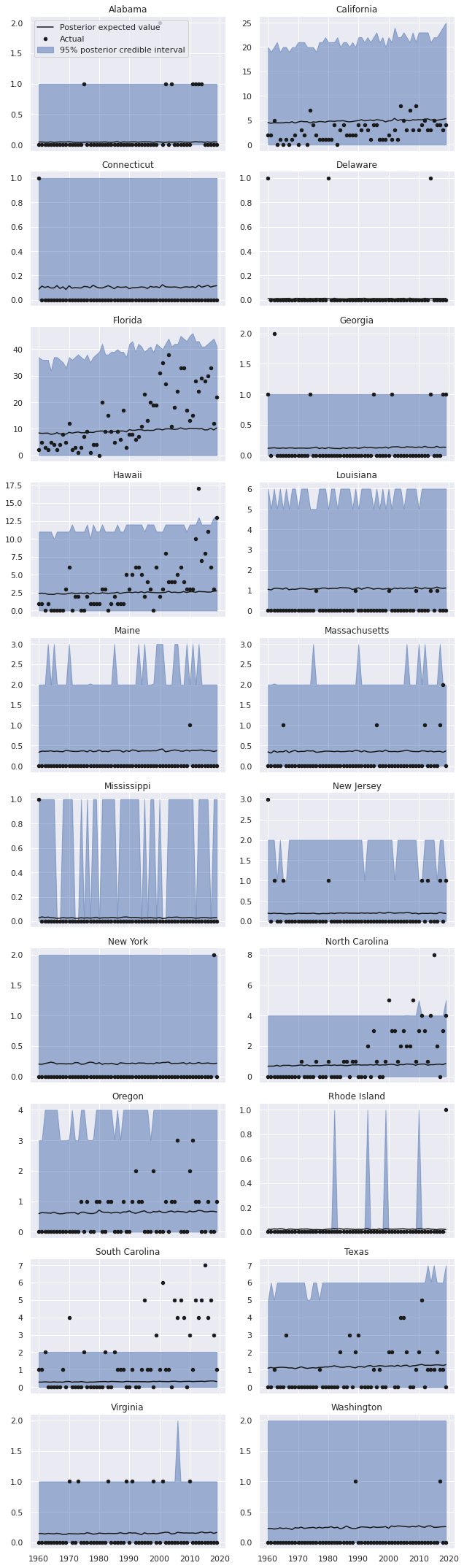nb_reg_pp_state_df = summarize_pp_df(nb_reg_pp_full_df, level="State")
ax = (nb_reg_pp_state_df.reset_index()
.plot.scatter("mean", "State", color='C0',
xerr=nb_reg_pp_state_df[["low", "high"]]
.sub(nb_reg_pp_state_df["mean"],
axis=0)
.abs()
.values.T,
zorder=5,
label="Posterior predictive mean"))
(attacks_df.reset_index()
.plot.scatter("Attacks", "State",
color='k', alpha=0.5,
label="Actual", ax=ax));

ax.set_xlabel("Number of shark Attacks");
ax.invert_yaxis();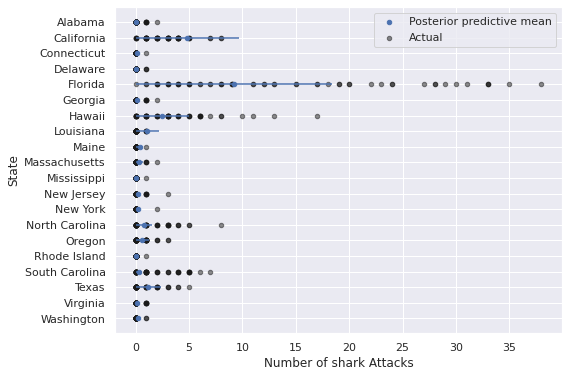These predictions certainly appear visually better than those from the regular negative binomial model without predictors, but still leave significant room for improvement, espescially in states like Florida, California, Hawaii, and the Carolinas.

We use Pareto-smoothed importance sampling leave-one-out cross-validation (PSIS-LOO) to quantify the improvement of this model over the basic negative binomial model.

traces = {
"NB": nb_trace,
"NB regression": nb_reg_trace
}
fig, ax = plt.subplots()

comp_df = az.compare(traces)
az.plot_compare(comp_df, plot_ic_diff=False, ax=ax);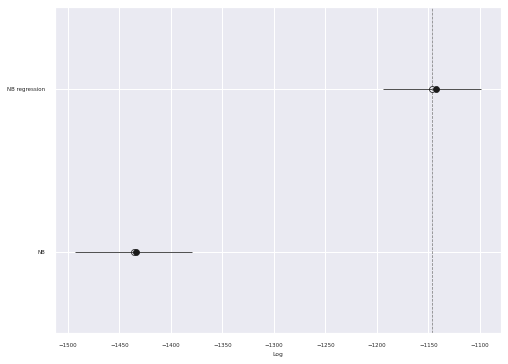We see that the PSIS-LOO score of the regression model is significantly higher than that of the simple negative binomial model.

### Mixed effects

There are many factors about each state that we have not included as predictors (average water temperature, prevalence of beach tourism, etc.). To account for this these and other unmeasured differences between states, we now add state-level mixed effects to our model.

Let $$j = 1, 2, \ldots, n_{\text{state}}$$ denote the index of a state and $$j(i)$$ denoth the index of the state of the $$i$$-th observation.

We place hierarchical normal priors on $$\beta_{0, j},$$ $$\beta_{\text{coast}, j},$$ and $$\beta_{\text{pop}, j}$$ and set

$\eta_{i, t} = \beta_{0, j(i)} + \beta_{\text{coast}, j(i)} \cdot x_{\text{coast}, i} + \beta_{\text{pop}, j(i)} \cdot x_{\text{pop}, i, t}.$ Again the mean is then $$\mu_{i, t} = \exp \eta_{i, t}.$$

The hierarchical prior for $$\beta_0$$ is equivalent to

\begin{align*} \mu_{\beta_0}, \gamma_{0, \text{pop}} & \sim N(0, 2.5^2) \\ \sigma_{\beta_0} & \sim \operatorname{Half}-N(2.5^2) \\ \beta_{0, j} & \sim N\left(\mu_{\beta_0} + \gamma_{0, \text{pop}} \cdot \bar{x}_{\text{pop}, j}, \sigma_{\beta_0}^2\right). \end{align*}

In reality, we implement this system of priors using a non-centered parametrization that is mathematically equivalent but often more computationally efficient. Note the inclusion of a term for $$\bar{x}_{\text{pop}, j}$$, which is the average population for the $$j$$-th state. We include this term to avoid violating the Gauss-Markov assumptions.

j_, state_map = (attacks_df.index
.get_level_values("State")
.factorize(sort=True))
n_state = state_map.size
j = at.constant(j_)
x_pop_bar = at.constant(standardize(
attacks_df.assign(x_pop=x_pop.eval())
.groupby(level="State")
["x_pop"]
.mean()
.values
))
def noncentered_normal(name, shape, μ=None):
if μ is None:
μ = pm.Normal(f"μ_{name}", 0., 2.5)

Δ = pm.Normal(f"Δ_{name}", 0., 1., shape=shape)
σ = pm.HalfNormal(f"σ_{name}", 2.5)

return pm.Deterministic(name, μ + Δ * σ)

def noncentered_normal_with_avg(name, avg_map, shape, μ=None):
if μ is None:
μ = pm.Normal(f"μ_{name}", 0., 2.5)

avg_terms_sum = 0.

for term_name, x_bar in avg_map.items():
avg_terms_sum += pm.Normal(f"γ_{name}_{term_name}_bar", 0., 2.5) * x_bar

return noncentered_normal(name, shape, μ=μ + avg_terms_sum)
with pm.Model() as mixed_model:
β0 = noncentered_normal_with_avg(
"β0", {"pop": x_pop_bar}, n_state
)

The prior for $$\beta_{\text{coast}, j}$$ is

\begin{align*} \mu_{\beta_\text{coast}}, \gamma_{\text{coast}^2}, \gamma_{\text{coast}, \text{pop}} & \sim N(0, 2.5^2) \\ \sigma_{\beta_{\text{coast}}} & \sim \operatorname{Half}-N(2.5^2) \\ \beta_{\text{coast}, j} & \sim N\left(\mu_{\beta_\text{coast}} + \gamma_{\text{coast}^2} \cdot x_{\text{coast}, j} + \gamma_{\text{coast}, \text{pop}} \cdot \bar{x}_{\text{pop}, j}, \sigma_{\beta_0}^2\right) \end{align*}

Here $$\bar{x}_{\text{coast}, j} = x_{\text{coast}, j}$$ since the a state’s coastline does not change over time (we ignoring the effects of global warming here). Due to this fact, we do not include the term $$\gamma_{0, \text{coast}} \cdot x_{\text{coast}, j}$$ in the definition of $$\beta_0$$, because this term would lead to $$\mu_{\beta_{\text{coast}}}$$ becoming unidentified. We use the analagous system of priors for $$\beta_{\text{pop}}.$$

log_state_coast = np.log(coast_df["Coastline"]
.loc[state_map]
.values)
x_coast_bar = at.constant(standardize(log_state_coast))
with mixed_model:
β_coast = noncentered_normal_with_avg(
"β_coast",
{"pop": x_pop_bar, "coast": x_coast_bar},
n_state
)
β_pop = noncentered_normal_with_avg(
"β_pop",
{"pop": x_pop_bar, "coast": x_coast_bar},
n_state)

The definitions of $$\eta_{i, t}$$, $$\mu_{i, t}$$, and $$\alpha$$ are similar to those in the previous model.

with mixed_model:
η = β0[j] + β_coast[j] * x_coast + β_pop[j] * x_pop
μ = at.exp(η)

α = pm.HalfNormal("α", 2.5)
obs = pm.NegativeBinomial("obs", μ, α, observed=y)

We again sample from the posterior distribution of the model.

with mixed_model:
mixed_trace = pm.sample(**SAMPLE_KWARGS, target_accept=0.95)
Auto-assigning NUTS sampler...
Multiprocess sampling (3 chains in 3 jobs)
NUTS: [μ_β0, γ_β0_pop_bar, Δ_β0, σ_β0, μ_β_coast, γ_β_coast_pop_bar, γ_β_coast_coast_bar, Δ_β_coast, σ_β_coast, μ_β_pop, γ_β_pop_pop_bar, γ_β_pop_coast_bar, Δ_β_pop, σ_β_pop, α]

100.00% [6000/6000 24:00<00:00 Sampling 3 chains, 3 divergences]

Sampling 3 chains for 1_000 tune and 1_000 draw iterations (3_000 + 3_000 draws total) took 1441 seconds.
There was 1 divergence after tuning. Increase target_accept or reparameterize.
There were 2 divergences after tuning. Increase target_accept or reparameterize.

The few divergences here could be eliminated by increasing target_accept and waiting longer, but we will proceed with our analysis (I’m impatient). As before, the standard sampling diagnostics show no cause for concern.

make_diagnostic_plots(mixed_trace);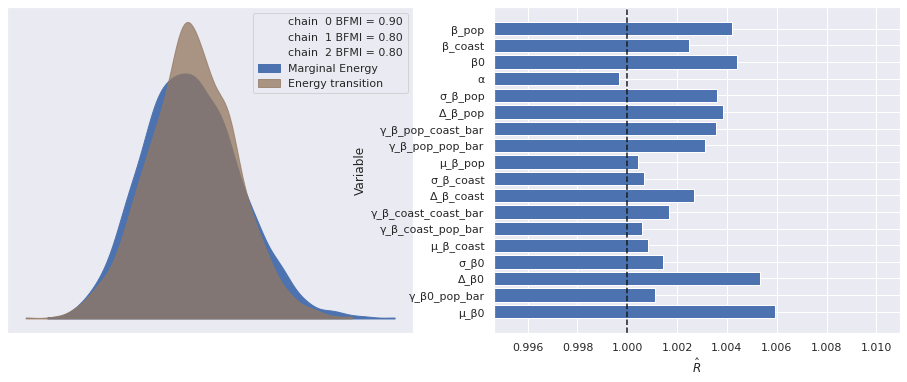To see if the inclusion of mixed effects has improved the model fit, we sample from and plot the posterior predictive distributions per state-year.

with mixed_model:
mixed_pp_trace = pm.sample_posterior_predictive(mixed_trace)

100.00% [3000/3000 00:05<00:00]

mixed_pp_full_df = make_pp_full_df(mixed_pp_trace["obs"])
mixed_pp_df = summarize_pp_df(mixed_pp_full_df)
grid = sns.FacetGrid(mixed_pp_df.reset_index(), col="State",
col_wrap=2, sharey=False, aspect=1.5)
grid.map_dataframe(plot_pp_over_time);

grid.axes.legend(loc="upper left");
grid.set_titles("{col_name}");
grid.fig.tight_layout();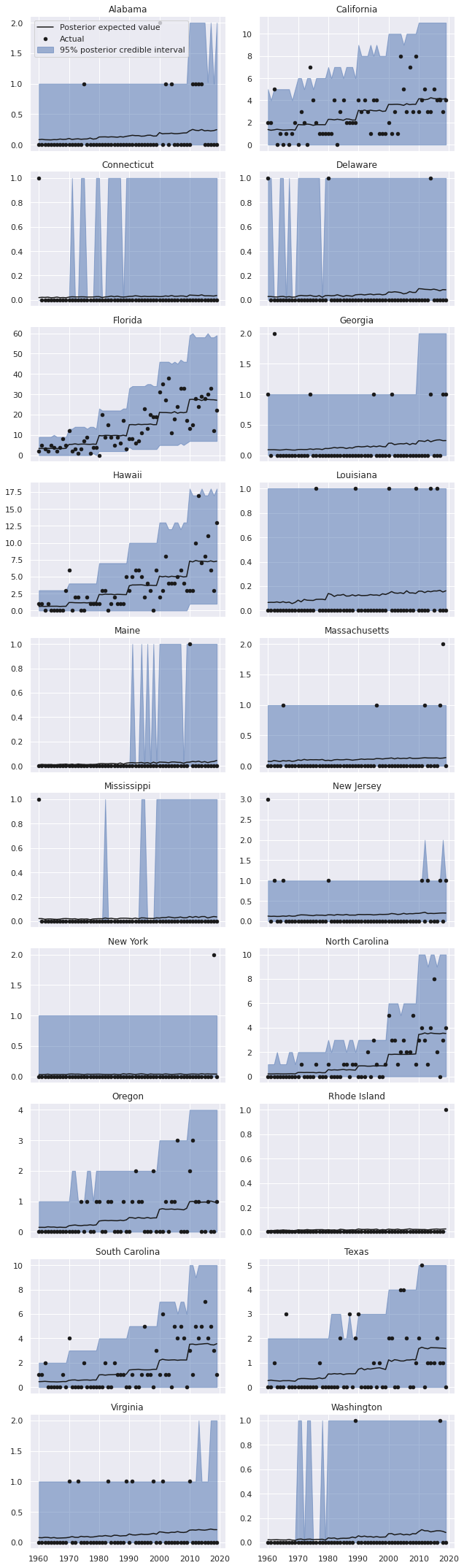mixed_pp_state_df = summarize_pp_df(mixed_pp_full_df, level="State")
ax = (mixed_pp_state_df.reset_index()
.plot.scatter("mean", "State", color='C0',
xerr=mixed_pp_state_df[["low", "high"]]
.sub(mixed_pp_state_df["mean"],
axis=0)
.abs()
.values.T,
zorder=5,
label="Posterior predictive mean"))
(attacks_df.reset_index()
.plot.scatter("Attacks", "State",
color='k', alpha=0.5,
label="Actual", ax=ax));

ax.set_xlabel("Number of shark Attacks");
ax.invert_yaxis();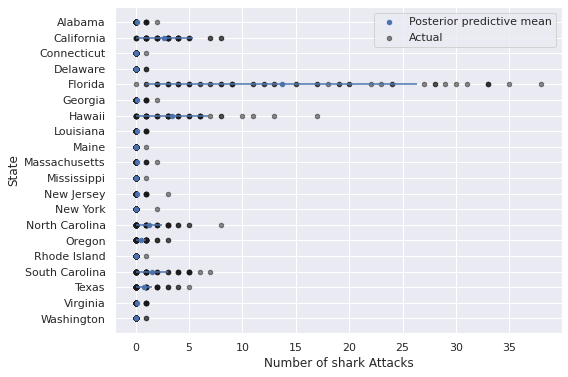We see that the predictions for Florida, California, Hawaii, and the Carolinas are much better in this model.

traces["NB mixed"] = mixed_trace
fig, ax = plt.subplots()

comp_df = az.compare(traces)
az.plot_compare(comp_df, plot_ic_diff=False, ax=ax);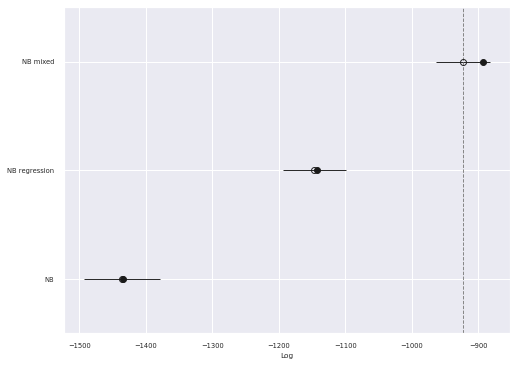As expected the PSIS-LOO score for this model is significantly better than the fixed effects negative binomial regression model.

It is interesting but not surprising that mixed effects are required to produce reasonable predictions of the number of shark attacks in each state over time. Future posts may investigate further explanatory variables and other aspects of this data set.

This post is available as a Jupyter notebook here.

%load_ext watermark
%watermark -n -u -v -iv
Last updated: Sun Jun 27 2021

Python implementation: CPython
Python version       : 3.8.8
IPython version      : 7.22.0

numpy     : 1.20.2
matplotlib: 3.4.1
pandas    : 1.2.3
us        : 2.0.2
seaborn   : 0.11.1
aesara    : 2.0.12
arviz     : 0.11.2
pymc3     : 4.0